## Graph functions using vertical and horizontal shifts

Often when given a problem, we try to model the scenario using mathematics in the form of words, tables, graphs, and equations. One method we can employ is to adapt the basic graphs of the toolkit functions to build new models for a given scenario. There are systematic ways to alter functions to construct appropriate models for the problems we are trying to solve.

## Identifying Vertical Shifts

One simple kind of transformation involves shifting the entire graph of a function up, down, right, or left. The simplest shift is a vertical shift, moving the graph up or down, because this transformation involves adding a positive or negative constant to the function. In other words, we add the same constant to the output value of the function regardless of the input. For a function $g\left(x\right)=f\left(x\right)+k$, the function $f\left(x\right)$ is shifted vertically $k$ units.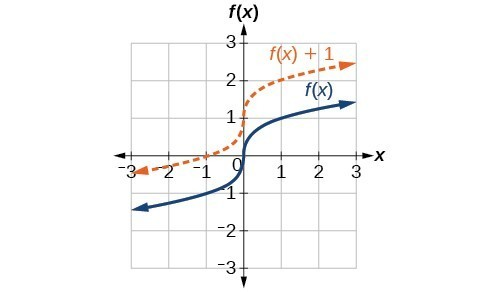Figure 2. Vertical shift by $k=1$ of the cube root function $f\left(x\right)=\sqrt{x}$.

To help you visualize the concept of a vertical shift, consider that $y=f\left(x\right)$. Therefore, $f\left(x\right)+k$ is equivalent to $y+k$. Every unit of $y$ is replaced by $y+k$, so the $y\text{-}$ value increases or decreases depending on the value of $k$. The result is a shift upward or downward.

### A General Note: Vertical Shift

Given a function $f\left(x\right)$, a new function $g\left(x\right)=f\left(x\right)+k$, where $k$ is a constant, is a vertical shift of the function $f\left(x\right)$. All the output values change by $k$ units. If $k$ is positive, the graph will shift up. If $k$ is negative, the graph will shift down.

### Example 1: Adding a Constant to a Function

To regulate temperature in a green building, airflow vents near the roof open and close throughout the day. Figure 2 shows the area of open vents $V$ (in square feet) throughout the day in hours after midnight, $t$. During the summer, the facilities manager decides to try to better regulate temperature by increasing the amount of open vents by 20 square feet throughout the day and night. Sketch a graph of this new function.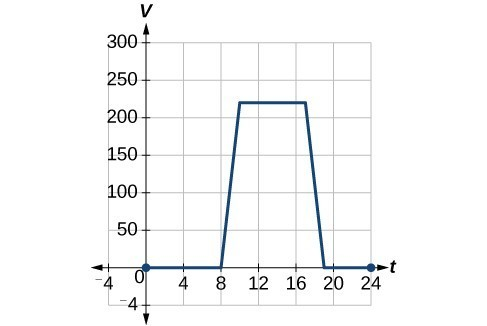Figure 3

### Solution

We can sketch a graph of this new function by adding 20 to each of the output values of the original function. This will have the effect of shifting the graph vertically up, as shown in Figure 4.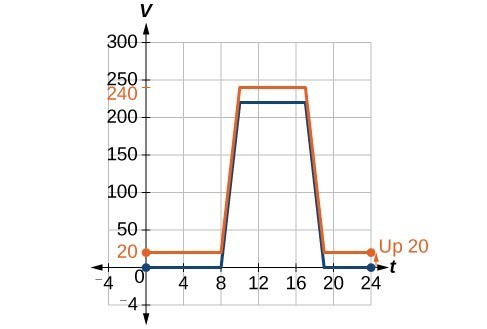Figure 4

Notice that for each input value, the output value has increased by 20, so if we call the new function $S\left(t\right)$, we could write

$S\left(t\right)=V\left(t\right)+20$

This notation tells us that, for any value of $t,S\left(t\right)$ can be found by evaluating the function $V$ at the same input and then adding 20 to the result. This defines $S$ as a transformation of the function $V$, in this case a vertical shift up 20 units. Notice that, with a vertical shift, the input values stay the same and only the output values change.

 $t$ 0 8 10 17 19 24 $V\left(t\right)$ 0 0 220 220 0 0 $S\left(t\right)$ 20 20 240 240 20 20

### How To: Given a tabular function, create a new row to represent a vertical shift.

1. Identify the output row or column.
2. Determine the magnitude of the shift.
3. Add the shift to the value in each output cell. Add a positive value for up or a negative value for down.

### Example 2: Shifting a Tabular Function Vertically

A function $f\left(x\right)$ is given below. Create a table for the function $g\left(x\right)=f\left(x\right)-3$.

 $x$ 2 4 6 8 $f\left(x\right)$ 1 3 7 11

### Solution

The formula $g\left(x\right)=f\left(x\right)-3$ tells us that we can find the output values of $g$ by subtracting 3 from the output values of $f$. For example:

$\begin{cases}f\left(2\right)=1\hfill & \text{Given}\hfill \\ g\left(x\right)=f\left(x\right)-3\hfill & \text{Given transformation}\hfill \\ g\left(2\right)=f\left(2\right)-3\hfill & \hfill \\ =1 - 3\hfill & \hfill \\ =-2\hfill & \hfill \end{cases}$

Subtracting 3 from each $f\left(x\right)$ value, we can complete a table of values for $g\left(x\right)$.

 $x$ 2 4 6 8 $f\left(x\right)$ 1 3 7 11 $g\left(x\right)$ −2 0 4 8

### Analysis of the Solution

As with the earlier vertical shift, notice the input values stay the same and only the output values change.

The function $h\left(t\right)=-4.9{t}^{2}+30t$ gives the height $h$ of a ball (in meters) thrown upward from the ground after $t$ seconds. Suppose the ball was instead thrown from the top of a 10-m building. Relate this new height function $b\left(t\right)$ to $h\left(t\right)$, and then find a formula for $b\left(t\right)$.

$b\left(t\right)=h\left(t\right)+10=-4.9{t}^{2}+30t+10$

## Identifying Horizontal Shifts

We just saw that the vertical shift is a change to the output, or outside, of the function. We will now look at how changes to input, on the inside of the function, change its graph and meaning. A shift to the input results in a movement of the graph of the function left or right in what is known as a horizontal shift.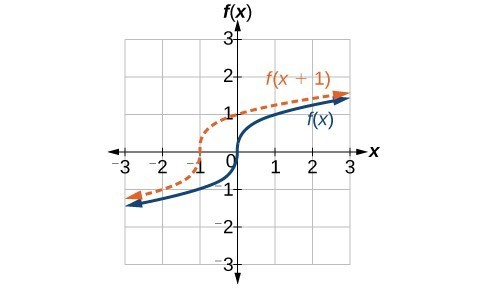Figure 5. Horizontal shift of the function $f\left(x\right)=\sqrt{x}$. Note that $h=+1$ shifts the graph to the left, that is, towards negative values of $x$.

For example, if $f\left(x\right)={x}^{2}$, then $g\left(x\right)={\left(x - 2\right)}^{2}$ is a new function. Each input is reduced by 2 prior to squaring the function. The result is that the graph is shifted 2 units to the right, because we would need to increase the prior input by 2 units to yield the same output value as given in $f$.

### A General Note: Horizontal Shift

Given a function $f$, a new function $g\left(x\right)=f\left(x-h\right)$, where $h$ is a constant, is a horizontal shift of the function $f$. If $h$ is positive, the graph will shift right. If $h$ is negative, the graph will shift left.

### Example 3: Adding a Constant to an Input

Returning to our building airflow example from Example 2, suppose that in autumn the facilities manager decides that the original venting plan starts too late, and wants to begin the entire venting program 2 hours earlier. Sketch a graph of the new function.

### Solution

We can set $V\left(t\right)$ to be the original program and $F\left(t\right)$ to be the revised program.

$\begin{cases}{c}V\left(t\right)=\text{ the original venting plan}\\ \text{F}\left(t\right)=\text{starting 2 hrs sooner}\end{cases}$

In the new graph, at each time, the airflow is the same as the original function $V$ was 2 hours later. For example, in the original function $V$, the airflow starts to change at 8 a.m., whereas for the function $F$, the airflow starts to change at 6 a.m. The comparable function values are $V\left(8\right)=F\left(6\right)$. Notice also that the vents first opened to $220{\text{ ft}}^{2}$ at 10 a.m. under the original plan, while under the new plan the vents reach $220{\text{ ft}}^{\text{2}}$ at 8 a.m., so $V\left(10\right)=F\left(8\right)$.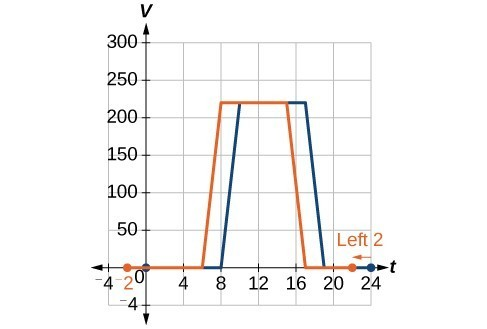Figure 6

In both cases, we see that, because $F\left(t\right)$ starts 2 hours sooner, $h=-2$. That means that the same output values are reached when $F\left(t\right)=V\left(t-\left(-2\right)\right)=V\left(t+2\right)$.

### Analysis of the Solution

Note that $V\left(t+2\right)$ has the effect of shifting the graph to the left.

Horizontal changes or “inside changes” affect the domain of a function (the input) instead of the range and often seem counterintuitive. The new function $F\left(t\right)$ uses the same outputs as $V\left(t\right)$, but matches those outputs to inputs 2 hours earlier than those of $V\left(t\right)$. Said another way, we must add 2 hours to the input of $V$ to find the corresponding output for $F:F\left(t\right)=V\left(t+2\right)$.

### How To: Given a tabular function, create a new row to represent a horizontal shift.

1. Identify the input row or column.
2. Determine the magnitude of the shift.
3. Add the shift to the value in each input cell.

### Example 4: Shifting a Tabular Function Horizontally

A function $f\left(x\right)$ is given below. Create a table for the function $g\left(x\right)=f\left(x - 3\right)$.

 $x$ 2 4 6 8 $f\left(x\right)$ 1 3 7 11

### Solution

The formula $g\left(x\right)=f\left(x - 3\right)$ tells us that the output values of $g$ are the same as the output value of $f$ when the input value is 3 less than the original value. For example, we know that $f\left(2\right)=1$. To get the same output from the function $g$, we will need an input value that is 3 larger. We input a value that is 3 larger for $g\left(x\right)$ because the function takes 3 away before evaluating the function $f$.

$\begin{cases}g\left(5\right)=f\left(5 - 3\right)\hfill \\ =f\left(2\right)\hfill \\ =1\hfill \end{cases}$

We continue with the other values to create this table.

 $x$ 5 7 9 11 $x - 3$ 2 4 6 8 $f\left(x\right)$ 1 3 7 11 $g\left(x\right)$ 1 3 7 11

The result is that the function $g\left(x\right)$ has been shifted to the right by 3. Notice the output values for $g\left(x\right)$ remain the same as the output values for $f\left(x\right)$, but the corresponding input values, $x$, have shifted to the right by 3. Specifically, 2 shifted to 5, 4 shifted to 7, 6 shifted to 9, and 8 shifted to 11.

### Analysis of the Solution

The graph in Figure 7 represents both of the functions. We can see the horizontal shift in each point.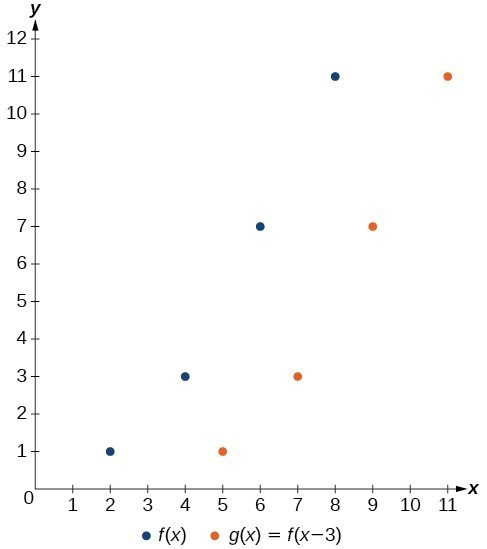Figure 7

### Example 5: Identifying a Horizontal Shift of a Toolkit Function

This graph represents a transformation of the toolkit function $f\left(x\right)={x}^{2}$. Relate this new function $g\left(x\right)$ to $f\left(x\right)$, and then find a formula for $g\left(x\right)$.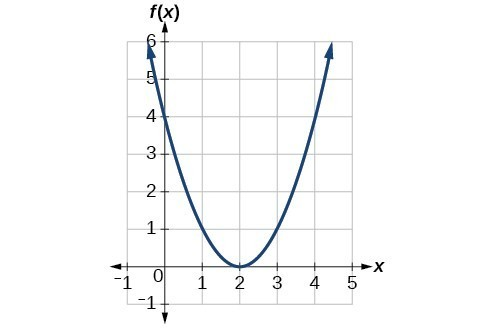Figure 8

### Solution

Notice that the graph is identical in shape to the $f\left(x\right)={x}^{2}$ function, but the x-values are shifted to the right 2 units. The vertex used to be at (0,0), but now the vertex is at (2,0). The graph is the basic quadratic function shifted 2 units to the right, so

$g\left(x\right)=f\left(x - 2\right)$

Notice how we must input the value $x=2$ to get the output value $y=0$; the x-values must be 2 units larger because of the shift to the right by 2 units. We can then use the definition of the $f\left(x\right)$ function to write a formula for $g\left(x\right)$ by evaluating $f\left(x - 2\right)$.

$\begin{cases}f\left(x\right)={x}^{2}\hfill \\ g\left(x\right)=f\left(x - 2\right)\hfill \\ g\left(x\right)=f\left(x - 2\right)={\left(x - 2\right)}^{2}\hfill \end{cases}$

### Analysis of the Solution

To determine whether the shift is $+2$ or $-2$ , consider a single reference point on the graph. For a quadratic, looking at the vertex point is convenient. In the original function, $f\left(0\right)=0$. In our shifted function, $g\left(2\right)=0$. To obtain the output value of 0 from the function $f$, we need to decide whether a plus or a minus sign will work to satisfy $g\left(2\right)=f\left(x - 2\right)=f\left(0\right)=0$. For this to work, we will need to subtract 2 units from our input values.

### Example 6: Interpreting Horizontal versus Vertical Shifts

The function $G\left(m\right)$ gives the number of gallons of gas required to drive $m$ miles. Interpret $G\left(m\right)+10$ and $G\left(m+10\right)$.

### Solution

$G\left(m\right)+10$ can be interpreted as adding 10 to the output, gallons. This is the gas required to drive $m$ miles, plus another 10 gallons of gas. The graph would indicate a vertical shift.

$G\left(m+10\right)$ can be interpreted as adding 10 to the input, miles. So this is the number of gallons of gas required to drive 10 miles more than $m$ miles. The graph would indicate a horizontal shift.

### Try It 1

Given the function $f\left(x\right)=\sqrt{x}$, graph the original function $f\left(x\right)$ and the transformation $g\left(x\right)=f\left(x+2\right)$ on the same axes. Is this a horizontal or a vertical shift? Which way is the graph shifted and by how many units?

Solution